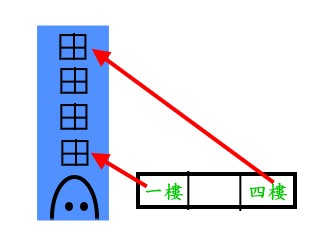• 其大小為4 bytes(假設硬體為32位元)
• 其內容存放2補數的整數數值
• 相關的運算符號有+,-,*(乘法),/,%,&(bitAND),|,^,~,<<,>>,=

• 其大小為4 bytes(假設硬體為32位元)
• 其內容存放記憶體的地址(可視為unsigned int)
• 相關的運算符號有*(透過pointer取記憶體內容),&(取變數地址),=,+,-&：取得變數的位址。

*：間接參考，指使用指標取得某個記憶體的內容。

int a=1, b=2, c;

int *p;   //宣告p是指向int的指標,英文說成p is a pointer to int

p = &a;  // &a是取a的地址(沒有&的話就變成取a的內容),=讓&a複製到p,也就是說現在p的內容為變數a的地址

b = *p;  //b的值被設定為指標p所指到記憶體的值(沒有*的話就變成取p的內容,而不是透過p去取a的內容了)

*p = 0;  //透過p將a的值被設定為0

p = &c;  //指標p現在指向c

*p = *p + 10;  // 透過p把某記憶體(現在是c)的值增加10

y = *p + 1;   //透過p取某記憶體的值(現在是c)加1之後，把結果存入y中

++*p; // 指標p所指到的記憶體上1,這是因為++和*優先權同樣為2,右結合,因此*先做,再做++

```#include <stdio.h>
int main() {
int x;
int *p;
p = 0; // 這讓p指到不合法的地方去了
*p = 0; // 透過p去設定不合法的記憶體, 會產生嚴重錯誤
x = *p; // 這也是錯的
}
```

```#include <stdio.h>
int main() {
int x;
int *p, *q;
p = x;
q = x + 20;
*(p+10) = 100; // 相當於x = 100
printf("offset between p and q is %d\", q - p); // 這會印出20
}
```

```#include <stdio.h>
main () {
float z = 5.5;
int x = 5;
int y = 5;
int *p;
p = &x;
p = p + 1; // 想把p指到哪裡去啊?
printf("%d %d %f\n", x, y, z);
*p = 20;
printf("%d %d %f\n", x, y, z);
}
```

```#include <stdio.h>
main () {
int y = 2;
int x = 5;
int *p;  // p is a pointer to int
int **q; // q is a pointer to pointer to integer
p = &x;  // 把p 指到 x
q = &p;  // 把 q 指到 p
*q = &y; // 透過q把p的內容改為指到y
printf("%d\n", *p); // 會印出2
printf("%d\n", **q); // 會印出2
}
```

### 傳遞指標

C語言的參數傳遞使用Call by Value,因此被呼叫的函數無法直接存取呼叫函數內的變數。若要達到類似Call by Reference的效果,就要透過傳遞指標來達成。

```void fun(int x, int y) {
x = 5
y = 5;
}
void main() {
int x = 0;
int y = 0;
fun(x, y);
printf("%d %d\n", x, y);
}
```

```void fun(int * x, int * y) {
*x = 5;
*y = 5;
}
void main() {
int x = 0;
int y = 0;
fun(&x, &y);
printf("%d %d\n", x, y);
}
```
fun裡的xy和main裡面的xy是完全不同的東西,一指標,一是整數,但fun裡的pointer x,y其內容是main裡x,y的地址,因此透過pointer x,y就可以去存取到int x,y了。